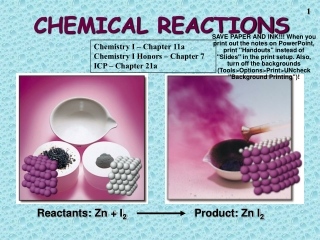DownloadDownload PresentationCHEMICAL REACTIONS

# CHEMICAL REACTIONS

Télécharger la présentation## CHEMICAL REACTIONS

- - - - - - - - - - - - - - - - - - - - - - - - - - - E N D - - - - - - - - - - - - - - - - - - - - - - - - - - -
##### Presentation Transcript

1. CHEMICAL REACTIONS SAVE PAPER AND INK!!! When you print out the notes on PowerPoint, print "Handouts" instead of "Slides" in the print setup. Also, turn off the backgrounds (Tools>Options>Print>UNcheck "Background Printing")! Chemistry I – Chapter 11aChemistry I Honors – Chapter 7ICP – Chapter 21a Reactants: Zn + I2 Product: Zn I2

2. Chemical Equations Their Job: Depict the kind of reactants and products and their relative amounts in a reaction. 4Al (s) + 3O2 (g) ---> 2Al2O3 (s) The numbers in the front are called stoichiometric ____________ The letters (s), (g), and (l) are the physical states of compounds.

3. Introduction • Chemical reactions occur when bonds between the outermost parts of atoms are formed or broken • Chemical reactions involve changes in matter, the making of new materials with new properties, and energy changes. • Symbols represent elements, formulas describe compounds, chemical equations describe a chemical reaction

4. Parts of a Reaction Equation • Chemical equations show the conversion of reactants (the molecules shown on the left of the arrow) into products (the molecules shown on the right of the arrow). • A + sign separates molecules on the same side • The arrow is read as “yields” • Example C + O2 CO2 • This reads “carbon plus oxygen react to yield carbon dioxide”

5. The charcoal used in a grill is basically carbon. The carbon reacts with oxygen to yield carbon dioxide. The chemical equation for this reaction, C + O2 CO2, contains the same information as the English sentence but has quantitative meaning as well.

6. Lavoisier, 1788 Chemical Equations Because of the principle of the conservation of matter, an equation must be balanced. It must have the same number of atoms of the same kind on both sides.

7. Symbols Used in Equations • Solid ___ • Liquid (l) • Gas ___ • Aqueous solution (aq) • Catalyst H2SO4 • Escaping gas () • Change of temperature ()

8. Balancing Equations • When balancing a chemical reaction you may add coefficients in front of the compounds to balance the reaction, but you may not change the subscripts. • Changing the subscripts changes the compound. Subscripts are determined by the valence electrons (charges for ionic or sharing for covalent)

9. Subscripts vs. Coefficients • The subscripts tell you how many atoms of a particular element are in a compound. The coefficient tells you about the quantity, or number, of molecules of the compound.

10. Chemical Equations 4 Al(s) + 3 O2(g) ---> 2 Al2O3(s) This equation means 4 Al atoms + 3 O2 molecules ---produces---> 2 molecules of Al2O3 AND/OR 4 moles of Al + 3 moles of O2 ---produces---> 2 moles of Al2O3

11. Steps to Balancing Equations There are four basic steps to balancing a chemical equation. • Write the correct formula for the reactants and the products. DO NOT TRY TO BALANCE IT YET! You must write the correct formulas first. And most importantly, once you write them correctly DO NOT CHANGE THE FORMULAS! • Find the number of atoms for each element on the left side. Compare those against the number of the atoms of the same element on the right side. • Determine where to place coefficients in front of formulas so that the left side has the same number of atoms as the right side for EACH element in order to balance the equation. • Check your answer to see if: • The numbers of atoms on both sides of the equation are now balanced. • The coefficients are in the lowest possible whole number ratios. (reduced)

12. Some Suggestions to Help You Some of Mr. Rapp’s Helpful Hints for balancing equations: • Take one element at a time, working left to right except for H and O. Save H for next to last, and O until last. • IF everything balances except for O, and there is no way to balance O with a whole number, double all the coefficients and try again. (Because O is diatomic as an element) • (Shortcut) Polyatomic ions that appear on both sides of the equation should be balanced as independent units

13. Balancing Equations 2 2 ___ H2(g) + ___ O2(g) ---> ___ H2O(l) What Happened to the Other Oxygen Atom????? This equation is not balanced! Two hydrogen atoms from a hydrogen molecule (H2) combines with one of the oxygen atoms from an oxygen molecule (O2) to form H2O. Then, the remaining oxygen atom combines with two more hydrogen atoms (from another H2 molecule) to make a second H2O molecule.

14. Balancing Equations ___ Al(s) + ___ Br2(l) ---> ___ Al2Br6(s) 2 3

15. Balancing Equations ____C3H8(g) + _____ O2(g) ----> _____CO2(g) + _____ H2O(g) ____B4H10(g) + _____ O2(g) ----> ___ B2O3(g) + _____ H2O(g)

16. Balancing Equations Sodium phosphate + iron (III) oxide  sodium oxide + iron (III) phosphate Na3PO4 + Fe2O3 ----> Na2O + FePO4